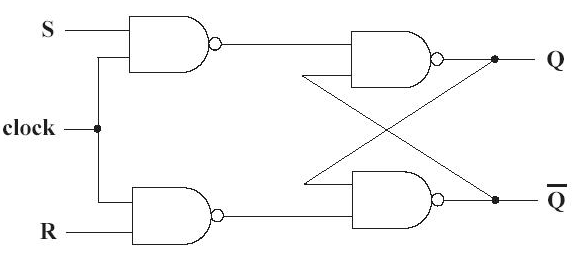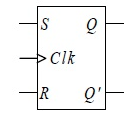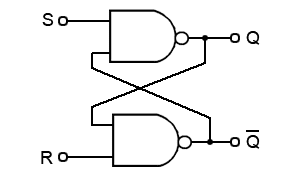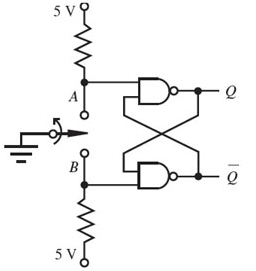# SR Flip Flop Explained in Detail

Flip – flops are one of the most fundamental electronic components. These are used as one-bit storage elements, clock dividers, and also we can make counters, shift registers and storing registers by connecting the flip flops in particular sequences. These flip flops use feedback concept to create sequential logic where the previous state affect future states (unlike combinational circuit).

The common types of flip flops are as follows:

SR (set-reset) flip flop is a sequential circuit consisting of two logic gates (mostly NAND or NOR gate). Here cross-coupling or positive feedback is formed. To achieve this we connect the output of each gate to the input of the other gate available. The storing bit present on the output with a label as Q.Symbol of SR Flip Flop

Now, one should not get a question that why the name is S-R flip only! are these some abbreviation? or initial of the inventor’s name?

No, ‘R’ and ‘S’ are simply the first letter of the two input names.

## Direct Coupled SR Flip Flop or SR Latch

It is a cross-coupled NAND or NOR gates as discussed earlier.

### NAND Gate Latch#### Truth Table

 S R Q Q’ 0 0 1 1(invalid) 0 1 1 0 1 0 0 1 1 1 0 1 1(Previous State) 0

We can see from the table that on giving active-low inputs (for both), both the outputs become active high (1) which is an invalid case. This is because Q and Q’ are complementary to each other. On the other hand, when both the inputs are active high,i.e., 1 then the output follows the previous state.

From the above discussion, we can note the following things about the basic bi-stable element.

1. The outputs Q & Q’ are complementary.
2. The circuit has two stable states. The state corresponds to Q=1 is referred to as 1 state or set state and corresponds to Q=0 is referred to as 0 state or reset state.
3. If the circuit is in set (1) state, it will remain in the set state & if the circuit is in reset (0) state, it will remain in the reset state. This property of the circuit shows that it can store 1-bit of digital information. Therefore, the circuit is called a 1-bit memory cell.
4. The 1-bit information stored in the circuit is locked or latched in the circuit. Therefore, this circuit is also referred to as a latch.

## Modified SR Latch

If in fig.1, two inverters are connected to enter the digital information and the inputs are S & R respectively then this latch is also called as SR latch.Fig: Modified circuit for SR latch

Consider the following cases:

### Case 1 : S = R = 0

In this case, S’ = R’ = 1. If Q is 1, Q and the other input for 2nd NAND gate are both 1 and hence Q’ = 0. Since, Q’ = 0 & S’ = 1, the output of 1st NAND gate is 1, Q = 1.

Similarly, calculate when Q = 0.

This shows that when S = R = 0, the outputs do not change.

### Case 2 : S = 1 and R =0

In this case, S’ = 0 and R’ = 1. Since S’ = 0, the output of 1st NAND gate, Q =1. For 2nd NAND gate, both inputs Q and R’ are 1, thus output Q’ = 0.

The inputs S=1 and R = 0, makes Q = 1, i.e., set state.

### Case 3 : S = 0 and R = 1

Since R’ = 0, the output of 2nd NAND gate, Q’ = 1. For 1st NAND gate, both inputs Q’ and S’ are 1, thus output Q = 0.

The inputs S = 0 and R = 1, makes Q = 0, i.e., reset state.

### Case 4 : S = 1 and R = 1

When S = R = 1, both the inputs Q and Q’ try to become 1 which is not allowed and therefore, this input condition is prohibited.

## Gated SR Latch

In the S R latch, we have seen that output changes occur immediately after the input changes occur i.e., the latch is sensitive to its S & R inputs at all times. However, it can easily be modified to create a latch that is sensitive to these inputs only when an enable input is active.Fig: SR latch with enable input (SR Flip Flop) using NAND gates

Below is the truth table where the circuit behaves like a S R latch when enable (EN = 1), and retains its previous state when EN = 0.

 EN S R Qn Qn+1 State 1 1 0 0 0 0 0 1 0 1 No Change (NC) 1 1 0 0 1 1 0 1 0 0 Reset 1 1 1 1 0 0 0 1 1 1 Set 1 1 1 1 1 1 0 1 X X Indeterminate 0 0 X X X X 0 1 0 1 No change (NC)

Table: Truth table for S R latch with enable input

## Excitation Table for SR Flip Flop

During the design process we get to know the sequence of states from the transition table, i.e., the transition from each present state to its corresponding next state. From this information we wish to find the flip flop input conditions that will cause the required transition. For this reason, we need a table that lists the required inputs for a given change of state. Such a table is known as an excitation table of the flip-flop.

We can derive the excitation tables for flip flops from their truth tables. The excitation table consists of two columns Qn and Qn+1 and a column for each input to show how the required transition can be achieved.

As shown in the table, there are four possible transitions from the present state to the next state. For each transition, the required input condition is derived from the information available in the truth table.

### SR Flip Flop Truth Table

 R S Qn+1 0 0 Qn 0 1 1 1 0 0 1 1 *

### SR Flip Flop Excitation Table

 Qn Qn+1 R S 0 0 X 0 0 1 0 1 1 0 1 0 1 1 0 X

#### 0 -> 0 Transition :

The present state of the flip flop is 0 and is to remain 0 when a clock pulse is applied. Looking at truth table of RS flip-flop  we can understand that, this can happen either when R = S = 0 (no change condition) or when R = 1 and S = 0. Thus, S has to be at 0, but R can be at either level. The table indicates this with a “0” under S and “X” (don’t care) under R.

#### 0->1 Transition :

The present state is 0 and is to change to 1. This can happen only when S = 1 and R = 0 (set condition). Therefore, S has to be 1 and R has to be 0 for this transition to occur.

#### 1-> 0 Transition :

The present state is 1 and is to change to a 0. This can happen only when S = 0 and R = 1(reset condition). Therefore,  S has to be 0 and R has to be 1 for this transition to occur.

#### 1-> 1 Transition :

The present state is 1 and is to remain 1. This can happen either when S = 1 and R = 0 (set condition) or when S = 0 and R = 0 (no change condition). Thus, R has to be 0, but S can be at either level. The table indicates this with a “X” under S and “0” under R.

### Problem in SR Flip Flop

There is a problem with this simple SR flip flop. From the truth table, we have seen a condition where the output becomes invalid when both S = R = 1.

Therefore, to overcome this issue, JK flip flop was developed.

## Applications of SR Flip Flop

Let us discuss the application of flip flop as a key debounce eliminator.

### Key Debounce

For interfacing keys to the digital systems, usually push button keys are used. These push button keys when pressed bounces a few times, closing and opening the contacts before proving a steady reading. Reading taken during bouncing period may be faulty. This problem is known as key debounce. The problem of key debounce is undesirable and it must be avoided.Fig: Switch debouncer

One way to avoid this is to use SR latch. The circuit used to avoid keybounce with SR latch is called a switch or contact debouncer. When key is at position A, the output of SR latch is logic 1, and when key is at position B, the output of SR latch is logic 0. It is important to note that, when key is in between A & B, SR inputs are 00 and hence output does not change, preventing debouncing of key output. In other words, we can say that the output does not change during transition period, eliminating key debounce.

AJAY DHEERAJ

(Technical Content Developer)

•
•
•
•
•
•